Metal sulphides and ammonia

Reaction scheme:

(Me = Metal, Cu or Zn)Precipitation:

The equilibrium reaction for an insoluble salt MZ is:

(MZ)s ó M+ + Z-

At equilibrium: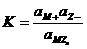For a solid a=1 therefore: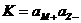The product of the activities of ions in a saturated solution is constant at a given temperatrure. This constant is called L (Löslichkeitsprodukt) or S (solubility product). For an ideal solution we can replace activities with concentrations:

S = [M+][Z-]

For convenience we calculate using concentrations although, in principle, we should check afterwards whether this is allowed. Only in ideal solutions we can use concentrations. For ZnS and CuS this means:

S = [Zn2+][S2-] = 2.93 10-25

S = [Cu2+][S2-] = 1.27 10-36

In pure water the concentration would be: [Zn2+]=[S2-]=Ö(2.93 10-25)= 5.4 10-13 M.

As can be seen in the reaction scheme presented above, the system we want to investigate is slightly more complex. If, for the moment, we ignore the protolysis of NH3 we would only have to consider the complex formation reaction of the Me2+ ions and the  NH3.

In other words:

Me2+ + NH3 ó Me(NH3)2+ + NH3 ó Me(NH3)22+ + NH3 ó Me(NH3)32+ + NH3 ó Me(NH3)42+

Whereby:Apart from these stability constants one can use the so-called gross=stability constants that are defined as: b1 = K1 and b2 = K1K2.  In reference works one usually finds the b-values but not the K-values.

Apart from the stability constants we also have to deal with the steuchiometric relations i.e. the gross-concentrations of Me2+ en NH3.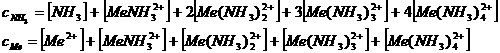However, NH3 is a protolyt, which means that: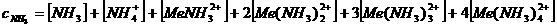To complete this set of equations we introduce:

As well as the electroneutrality equation: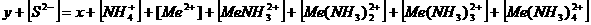So far we have only been formulating equations. However, it should be clear that the solubility of a metal sulphide can be increased significantly by complex formation with ammonia. The concentration of ammonia is therefore an important factor. That concentration is influenced by the pH.

If we want to quantify the influence of pH and NH3 concentration on the solubility of a metal sulphide we have to rearrange the solubility equation. This means that we have to express [Me2+] in terms of pH en [NH3]. We assume that S2- does not react further: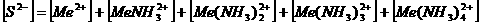withfor the solubility equations we get:The problem with the equation presented above that pH dependency is not incorporated. However, with:and with: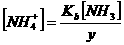we get:From this we have to derive an equation that expresses  [NH3] in terms of K’s, y, and cNH3.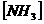= ……

This equation is than plugged into: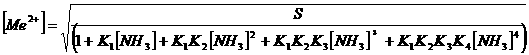With the resulting equation one can investigate the influence of pH and ammonia concentration (cNH3) on the solubility of metal sulphides.

Unfortunately, solving such a polynomial equation analytically is rather complicated. However, this problem can be circumvented by employing goalseek in excel.

The actual NH3 concentration can be calculated for a series of “gross” NH3 concentration and pH as is shown in the Table and Figure below.

The calculations are based on ZnS as that is the “more” soluble compound.

Table 1 : [NH3] as a function of CNH3 and pH for ZnS
(all concentrations in M)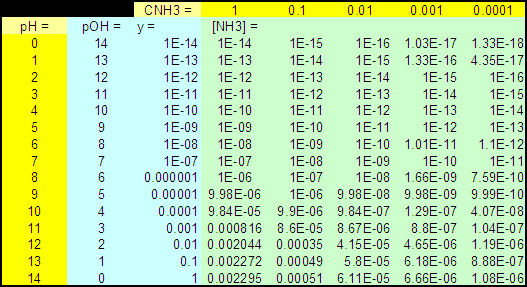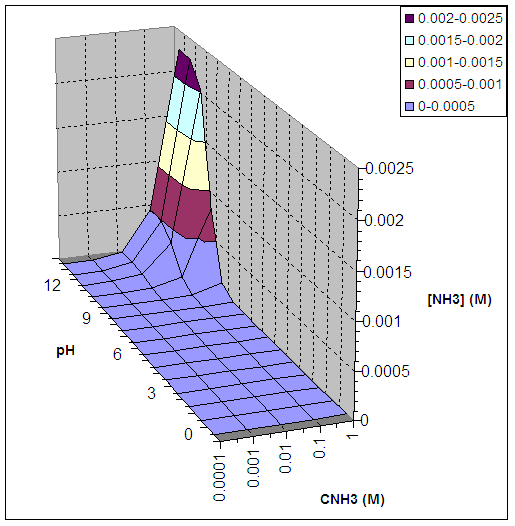Figure 1 : [NH3] as a function of CNH3 and pH for ZnS

As can be seen in the graph and table, in this environment NH3 is only present at high pH and high “gross” ammonia concentration.

For each “grid point” in the graph the ammonia concentration is know thereby making it possible to calculate the concentration of each metal-ion species in the solution, i.e. Me2+, Me(NH3)2+ , Me(NH3)22+ , Me(NH3)32+ and Me(NH3)42+ using the equations derived above. These concentrations are then summarized to calculate the total metal-ion species concentration. This total metal-ion species concentration is the same as the sulphur ion concentration in solution.

The results are shown in the table and graph below.

Table 2 : Total Metal ion concentration as a function of CNH3 and pH for ZnS
(all concentrations in M)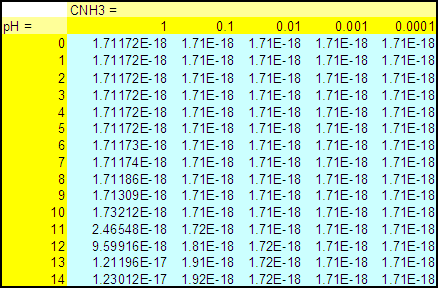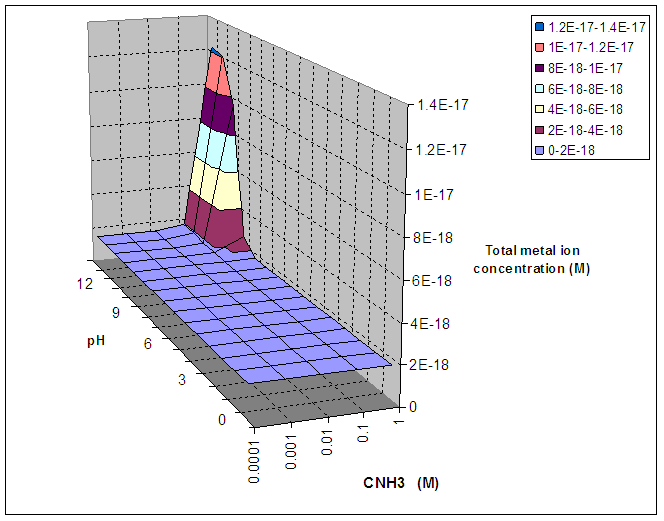Figure 2 : Total Metal ion concentration as a function of CNH3 and pH for ZnS

As can be seen in the graph and table above the highest total metal ion i.e. sulphur ion concentration found is 1.23 10-17 M at CNH3 = 1 M.